Скачать презентацию 1 Теория Информации В. Н. Ярмолик Лекция 3

it_lec_3.ppt

• Размер: 1.4 Mегабайта
• Количество слайдов: 18

## Описание презентации 1 Теория Информации В. Н. Ярмолик Лекция 3 по слайдам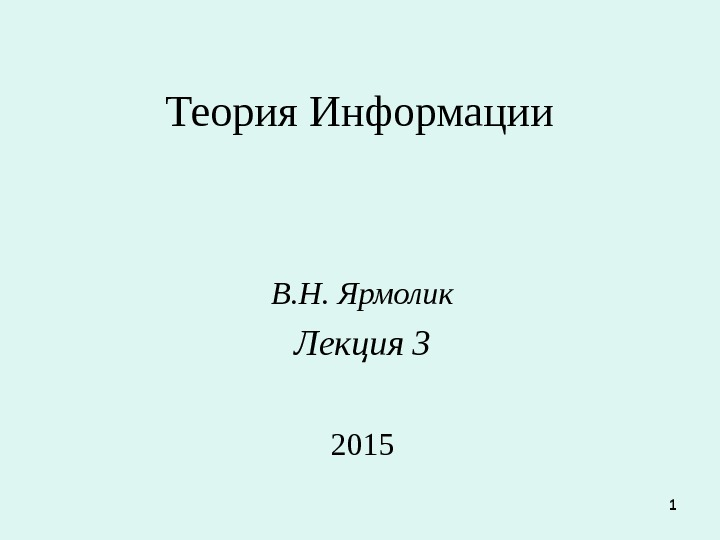1 Теория Информации В. Н. Ярмолик Лекция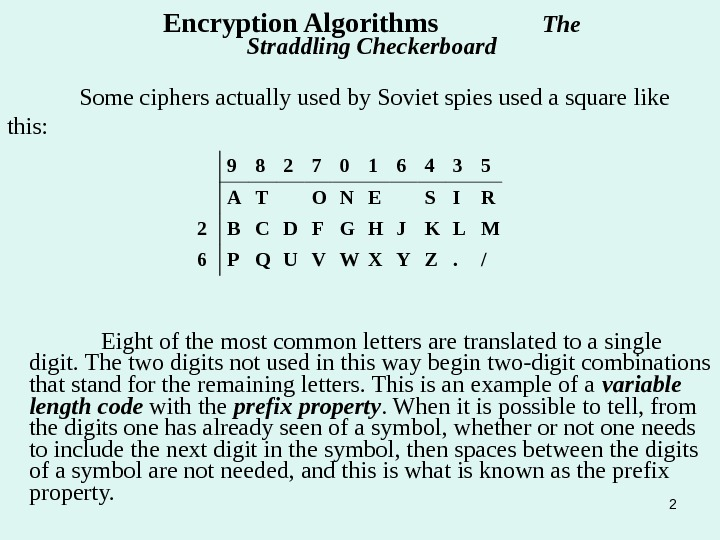2 Some ciphers actually used by Soviet spies used a square like this: Encryption Algorithms The Straddling Checkerboard 9 8 2 7 0 1 6 4 3 5 A T O N E S I R 2 B C D F G H J K L M 6 P Q U V W X Y Z. / Eight of the most common letters are translated to a single digit. The two digits not used in this way begin two-digit combinations that stand for the remaining letters. This is an example of a variable length code with the prefix property. When it is possible to tell, from the digits one has already seen of a symbol, whether or not one needs to include the next digit in the symbol, then spaces between the digits of a symbol are not needed, and this is what is known as the prefix property.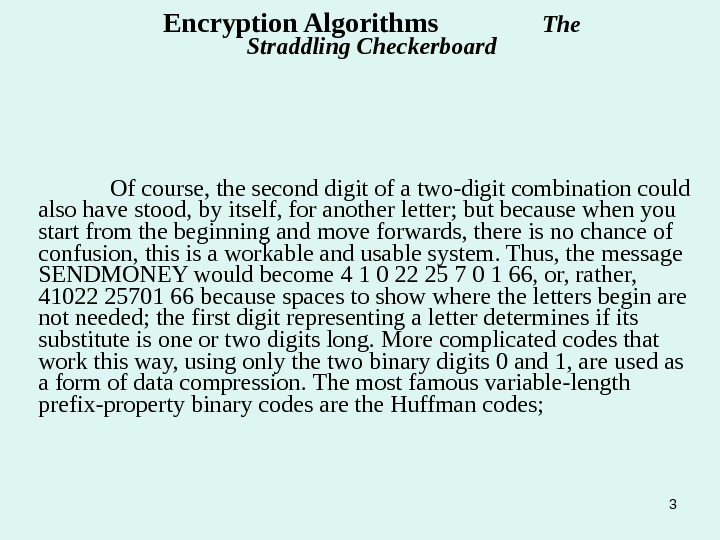3 Of course, the second digit of a two-digit combination could also have stood, by itself, for another letter; but because when you start from the beginning and move forwards, there is no chance of confusion, this is a workable and usable system. Thus, the message SENDMONEY would become 4 1 0 22 25 7 0 1 66, or, rather, 41022 25701 66 because spaces to show where the letters begin are not needed; the first digit representing a letter determines if its substitute is one or two digits long. More complicated codes that work this way, using only the two binary digits 0 and 1, are used as a form of data compression. The most famous variable-length prefix-property binary codes are the Huffman codes; Encryption Algorithms The Straddling Checkerboard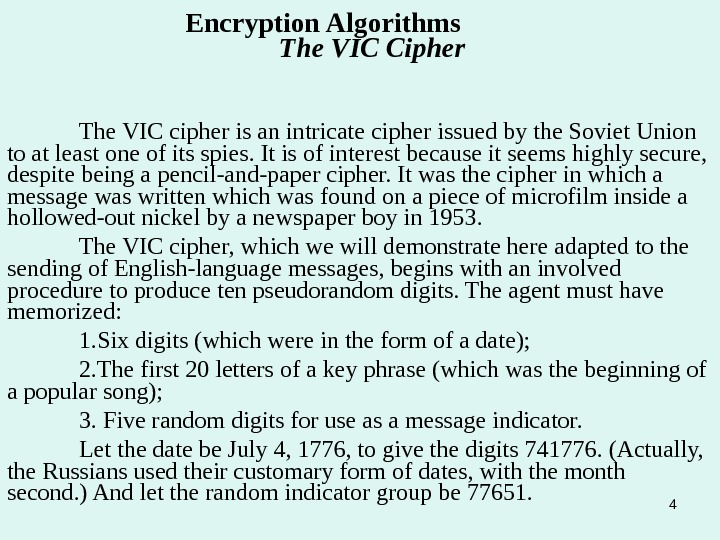4 The VIC cipher is an intricate cipher issued by the Soviet Union to at least one of its spies. It is of interest because it seems highly secure, despite being a pencil-and-paper cipher. It was the cipher in which a message was written which was found on a piece of microfilm inside a hollowed-out nickel by a newspaper boy in 1953. The VIC cipher, which we will demonstrate here adapted to the sending of English-language messages, begins with an involved procedure to produce ten pseudorandom digits. The agent must have memorized: 1. Six digits (which were in the form of a date); 2. The first 20 letters of a key phrase (which was the beginning of a popular song); 3. Five random digits for use as a message indicator. Let the date be July 4, 1776, to give the digits 741776. (Actually, the Russians used their customary form of dates, with the month second. ) And let the random indicator group be 77651. Encryption Algorithms The VIC Cipher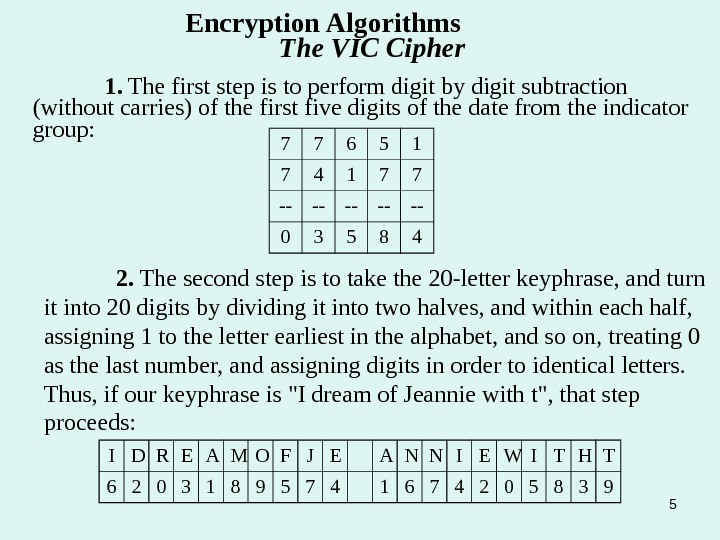51. The first step is to perform digit by digit subtraction (without carries) of the first five digits of the date from the indicator group: 2. The second step is to take the 20 -letter keyphrase, and turn it into 20 digits by dividing it into two halves, and within each half, assigning 1 to the letter earliest in the alphabet, and so on, treating 0 as the last number, and assigning digits in order to identical letters. Thus, if our keyphrase is «I dream of Jeannie with t», that step proceeds: Encryption Algorithms The VIC Cipher 7 7 6 5 1 7 4 1 7 7 — — — 0 3 5 8 4 I D R E A M O F J E A N N I E W I T H T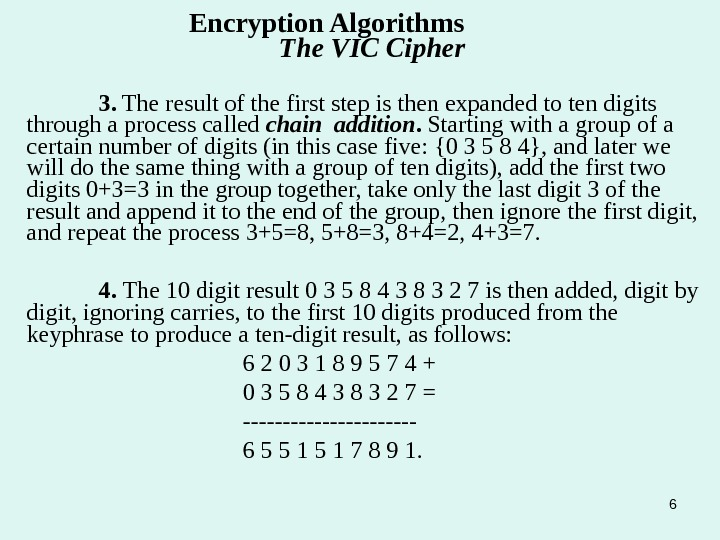6 Encryption Algorithms The VIC Cipher 3. The result of the first step is then expanded to ten digits through a process called chain addition. Starting with a group of a certain number of digits (in this case five: {0 3 5 8 4}, and later we will do the same thing with a group of ten digits), add the first two digits 0+3=3 in the group together, take only the last digit 3 of the result and append it to the end of the group, then ignore the first digit, and repeat the process 3+5=8, 5+8=3, 8+4=2, 4+3=7. 4. The 10 digit result 0 3 5 8 4 3 8 3 2 7 is then added, digit by digit, ignoring carries, to the first 10 digits produced from the keyphrase to produce a ten-digit result, as follows: 6 2 0 3 1 8 9 5 7 4 + 0 3 5 8 4 3 8 3 2 7 = ———— 6 5 5 1 7 8 9 1.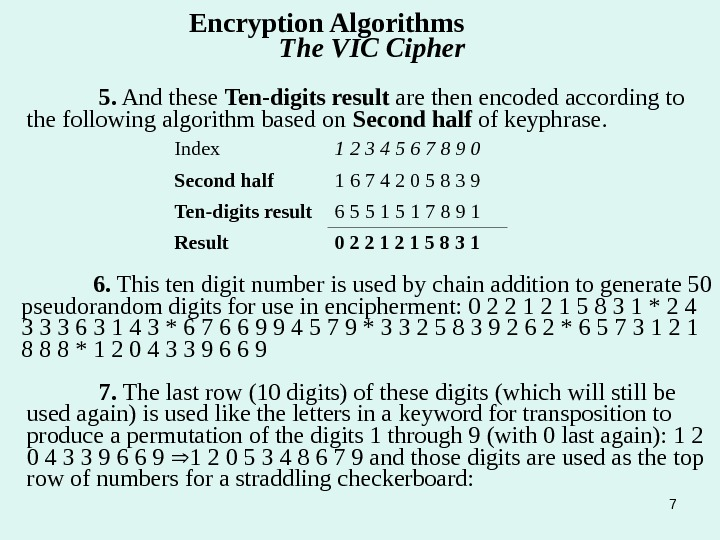75. And these Ten-digits result are then encoded according to the following algorithm based on Second half of keyphrase. Index 1 2 3 4 5 6 7 8 9 0 Second half 1 6 7 4 2 0 5 8 3 9 Ten-digits result 6 5 5 1 7 8 9 1 Result 0 2 2 1 5 8 3 1 6. This ten digit number is used by chain addition to generate 50 pseudorandom digits for use in encipherment: 0 2 2 1 5 8 3 1 * 2 4 3 3 3 6 3 1 4 3 * 6 7 6 6 9 9 4 5 7 9 * 3 3 2 5 8 3 9 2 6 2 * 6 5 7 3 1 2 1 8 8 8 * 1 2 0 4 3 3 9 6 6 9 7. The last row (10 digits) of these digits (which will still be used again) is used like the letters in a keyword for transposition to produce a permutation of the digits 1 through 9 (with 0 last again): 1 2 0 4 3 3 9 6 6 9 1 2 0 5 3 4 8 6 7 9 and those digits are used as the top row of numbers for a straddling checkerboard: Encryption Algorithms The VIC Cipher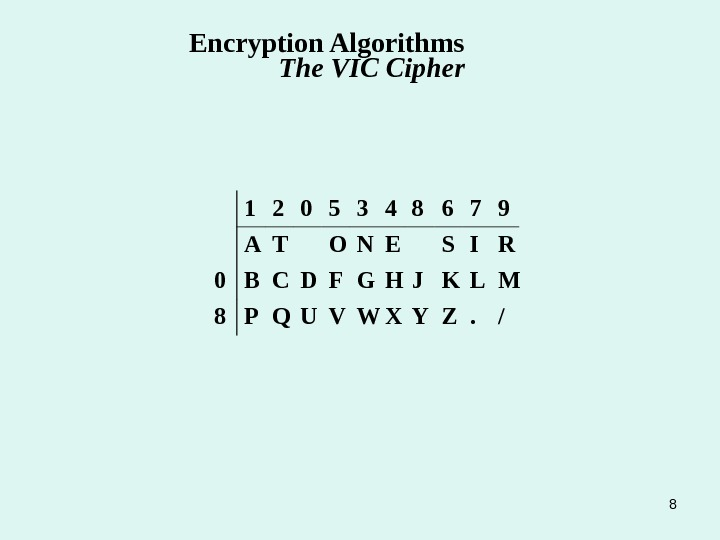81 2 0 5 3 4 8 6 7 9 A T O N E S I R 0 B C D F G H J K L M 8 P Q U V W X Y Z. /Encryption Algorithms The VIC Cipher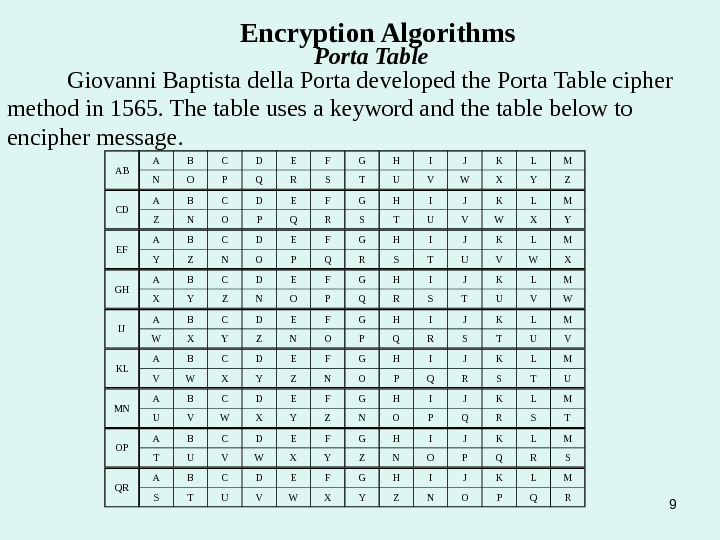9 Encryption Algorithms Porta Table Giovanni Baptista della Porta developed the Porta Table cipher method in 1565. The table uses a keyword and the table below to encipher message. AB A B C D E F G H I J K L M N O P Q R S T U V W X Y Z CD A B C D E F G H I J K L M Z N O P Q R S T U V W X Y EF A B C D E F G H I J K L M Y Z N O P Q R S T U V W X GH A B C D E F G H I J K L M X Y Z N O P Q R S T U V W IJ A B C D E F G H I J K L M W X Y Z N O P Q R S T U V KL A B C D E F G H I J K L M V W X Y Z N O P Q R S T U MN A B C D E F G H I J K L M U V W X Y Z N O P Q R S T OP A B C D E F G H I J K L M T U V W X Y Z N O P Q R S QR A B C D E F G H I J K L M S T U V W X Y Z N O P Q R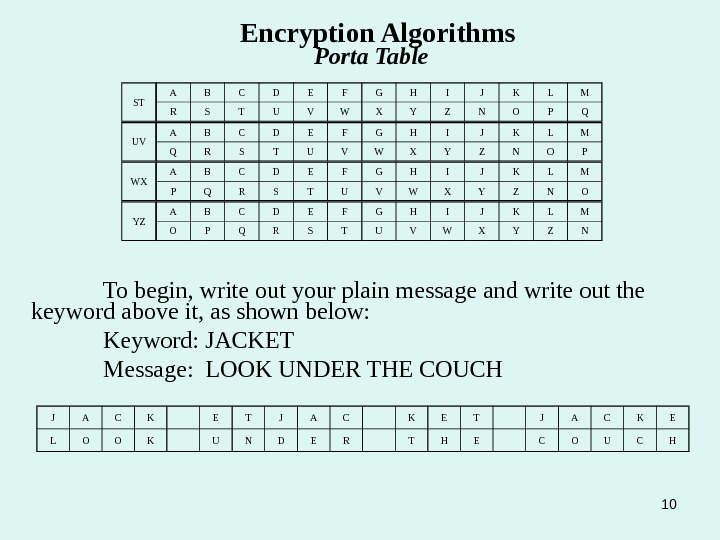10 ST A B C D E F G H I J K L M R S T U V W X Y Z N O P Q Encryption Algorithms Porta Table UV A B C D E F G H I J K L M Q R S T U V W X Y Z N O P WX A B C D E F G H I J K L M P Q R S T U V W X Y Z N O YZ A B C D E F G H I J K L M O P Q R S T U V W X Y Z N To begin, write out your plain message and write out the keyword above it, as shown below: Keyword: JACKET Message: LOOK UNDER THE COUCH J A C K E T J A C K E L O O K U N D E R T H E C O U C H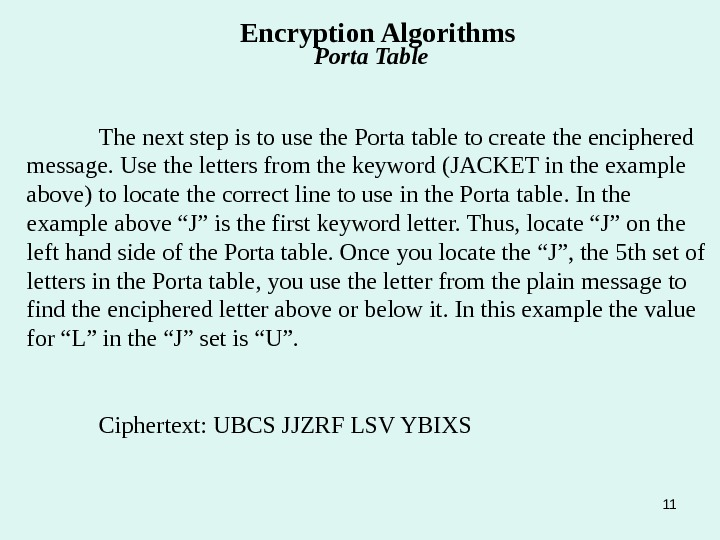11 The next step is to use the Porta table to create the enciphered message. Use the letters from the keyword (JACKET in the example above) to locate the correct line to use in the Porta table. In the example above “J” is the first keyword letter. Thus, locate “J” on the left hand side of the Porta table. Once you locate the “J”, the 5 th set of letters in the Porta table, you use the letter from the plain message to find the enciphered letter above or below it. In this example the value for “L” in the “J” set is “U”. Ciphertext: UBCS JJZRF LSV YBIXS Encryption Algorithms Porta Table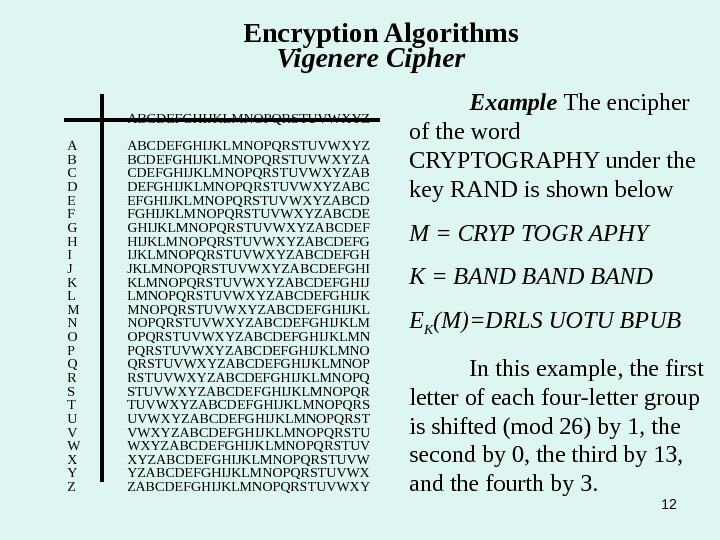12 Encryption Algorithms Vigenere Cipher ABCDEFGHIJKLMNOPQRSTUVWXYZ A ABCDEFGHIJKLMNOPQRSTUVWXYZ B BCDEFGHIJKLMNOPQRSTUVWXYZA C CDEFGHIJKLMNOPQRSTUVWXYZAB D DEFGHIJKLMNOPQRSTUVWXYZABC E EFGHIJKLMNOPQRSTUVWXYZABCD F FGHIJKLMNOPQRSTUVWXYZABCDE G GHIJKLMNOPQRSTUVWXYZABCDEF H HIJKLMNOPQRSTUVWXYZABCDEFG I IJKLMNOPQRSTUVWXYZABCDEFGH J JKLMNOPQRSTUVWXYZABCDEFGHI K KLMNOPQRSTUVWXYZABCDEFGHIJ L LMNOPQRSTUVWXYZABCDEFGHIJK M MNOPQRSTUVWXYZABCDEFGHIJKL N NOPQRSTUVWXYZABCDEFGHIJKLM O OPQRSTUVWXYZABCDEFGHIJKLMN P PQRSTUVWXYZABCDEFGHIJKLMNO Q QRSTUVWXYZABCDEFGHIJKLMNOP R RSTUVWXYZABCDEFGHIJKLMNOPQ S STUVWXYZABCDEFGHIJKLMNOPQR T TUVWXYZABCDEFGHIJKLMNOPQRS U UVWXYZABCDEFGHIJKLMNOPQRST V VWXYZABCDEFGHIJKLMNOPQRSTU W WXYZABCDEFGHIJKLMNOPQRSTUV X XYZABCDEFGHIJKLMNOPQRSTUVW Y YZABCDEFGHIJKLMNOPQRSTUVWX Z ZABCDEFGHIJKLMNOPQRSTUVWXY Example The encipher of the word CRYPTOGRAPHY under the key RAND is shown below M = CRYP TOGR APHY K = BAND E K (M)=DRLS UOTU BPUB In this example, the first letter of each four-letter group is shifted (mod 26) by 1, the second by 0, the third by 13, and the fourth by 3.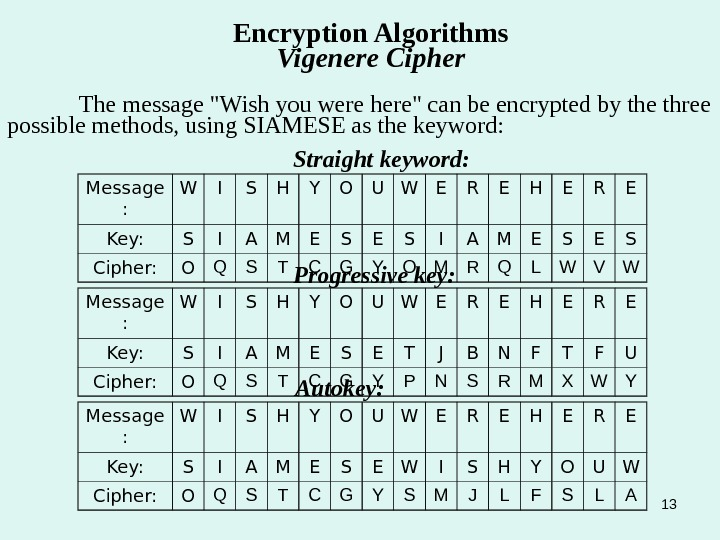13 Encryption Algorithms Vigenere Cipher The message «Wish you were here» can be encrypted by the three possible methods, using SIAMESE as the keyword: Straight keyword: Message : W I S H Y O U W E R E H E R E Key: S I A M E S E S Cipher: O Q S T C G Y O M R Q L W V W Progressive key: Message : W I S H Y O U W E R E H E R E Key: S I A M E S E T J B N F T F U Cipher: O Q S T C G Y P N S R M X W Y Autokey: Message : W I S H Y O U W E R E H E R E Key: S I A M E S E W I S H Y O U W Cipher: O Q S T C G Y S M J L F S L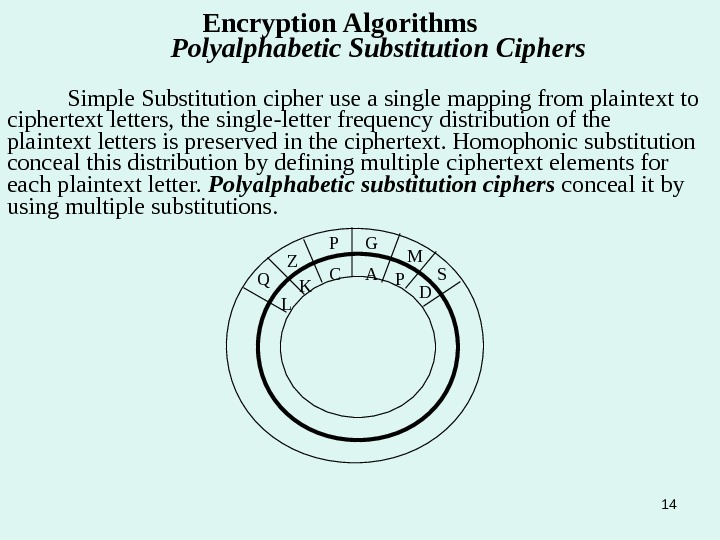14 Encryption Algorithms Polyalphabetic Substitution Ciphers Simple Substitution cipher use a single mapping from plaintext to ciphertext letters, the single-letter frequency distribution of the plaintext letters is preserved in the ciphertext. Homophonic substitution conceal this distribution by defining multiple ciphertext elements for each plaintext letter. Polyalphabetic substitution ciphers conceal it by using multiple substitutions. AGP C DM LQ Z S K P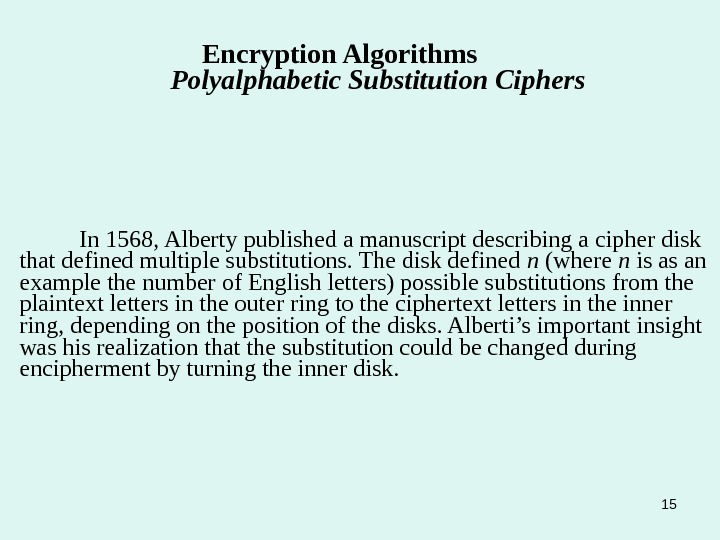15 In 1568, Alberty published a manuscript describing a cipher disk that defined multiple substitutions. The disk defined n (where n is as an example the number of English letters) possible substitutions from the plaintext letters in the outer ring to the ciphertext letters in the inner ring, depending on the position of the disks. Alberti’s important insight was his realization that the substitution could be changed during encipherment by turning the inner disk. Encryption Algorithms Polyalphabetic Substitution Ciphers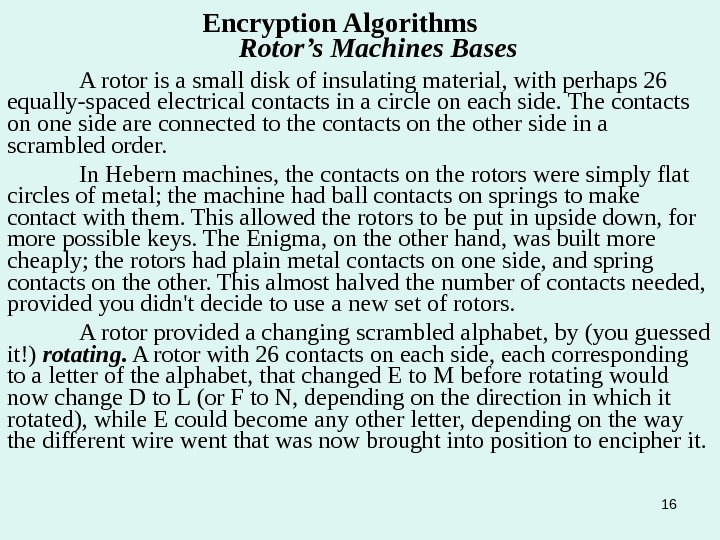16 Encryption Algorithms Rotor’s Machines Bases A rotor is a small disk of insulating material, with perhaps 26 equally-spaced electrical contacts in a circle on each side. The contacts on one side are connected to the contacts on the other side in a scrambled order. In Hebern machines, the contacts on the rotors were simply flat circles of metal; the machine had ball contacts on springs to make contact with them. This allowed the rotors to be put in upside down, for more possible keys. The Enigma, on the other hand, was built more cheaply; the rotors had plain metal contacts on one side, and spring contacts on the other. This almost halved the number of contacts needed, provided you didn’t decide to use a new set of rotors. A rotor provided a changing scrambled alphabet, by (you guessed it!) rotating. A rotor with 26 contacts on each side, each corresponding to a letter of the alphabet, that changed E to M before rotating would now change D to L (or F to N, depending on the direction in which it rotated), while E could become any other letter, depending on the way the different wire went that was now brought into position to encipher it.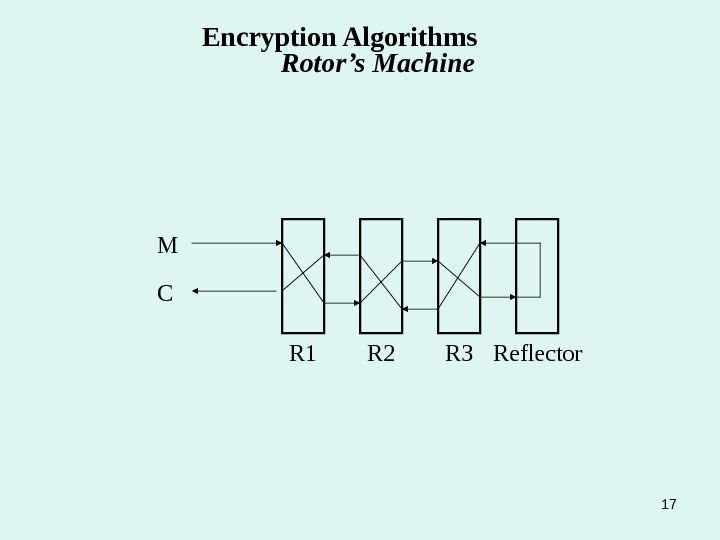17 R 3 R 2 R 1 Reflector. CM Encryption Algorithms Rotor’s Machine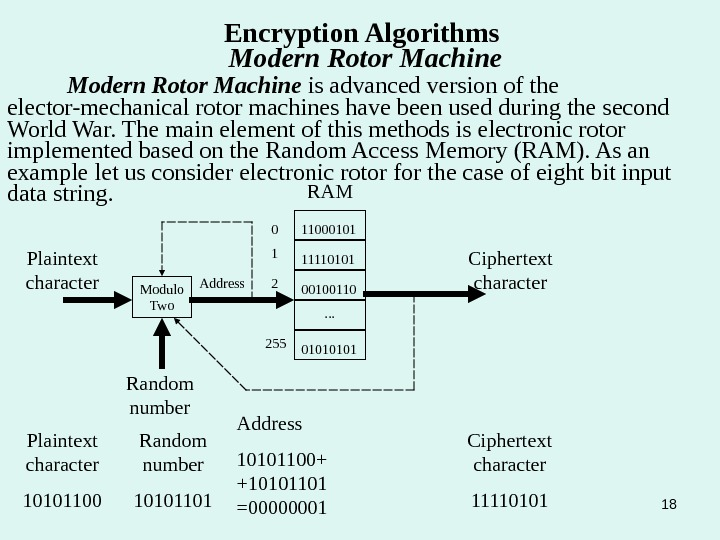18 Encryption Algorithms Modern Rotor Machine is advanced version of the elector-mechanical rotor machines have been used during the second World War. The main element of this methods is electronic rotor implemented based on the Random Access Memory (RAM). As an example let us consider electronic rotor for the case of eight bit input data string. . 1 001001101111010111000101 RAM 0 2 255 Modulo Two Address. Plaintext character Random number Ciphertext character 0101 Plaintext character 10101100 Random number 10101101 Address 10101100+ +10101101 =00000001 Ciphertext character